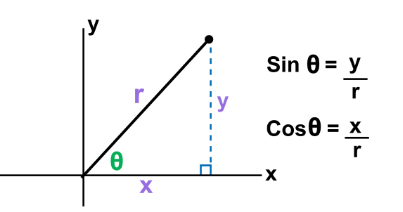# Cofunctions Worksheets

What Are Cofunctions? Cofunctions in trigonometry are function pairs like sine and cosine. a function f is cofunction of a function g if f(A) = g(B) whenever A and B are complementary angles. In Math, the two functions which are similar to each other are called cofunctions of each other as they are formed using the same angle. These functions complement each other. Well, in any triangle, we know the sum of the interior angles equals 180 degrees. In a right triangle, one of the angles is 90 degrees. Therefore, there are 90 degrees left between the other two angles. So, the sum of the other two angles must equal 90 degrees – so we say these two angles must be complementary!The graphical representation above shows the geometric representation of cofunctions.

• ### Basic Lesson

Guides students solving equations that involve an Cofunctions. Demonstrates answer checking.

• ### Intermediate Lesson

Demonstrates how to solve more difficult problems.

• ### Independent Practice 1

A really great activity for allowing students to understand the concept of cofunctions.

• ### Independent Practice 2

Students find the cofunctions in assorted problems. The answers can be found below.

• ### Homework Worksheet

Students are provided with problems to achieve the concepts of cofunctions.

• ### Skill Quiz

This tests the students ability to evaluate math statements with cofunctions.